# AI MACHINE LEARNING: BAGGING LINEAR FIT BOOTSTRAP (SUPERVISED)

• Related AI/ML Methods: Bagging Nonlinear Fit Bootstrap, Ensemble Common & Complex Fit
• Related Traditional Methods: Nonparametric Bootstrap Simulation, Bootstrap Regression

This method applies a Bootstrap Aggregation (Bagging) Linear Fit Model of hundreds of models via resampled data to generate the best consensus forecasts. The idea is that in a random selection of data, taking the average forecast of an ensemble of models provides a more accurate prediction than a single sample. In a typical multivariate linear regression, the relationship structure and characteristics of a dependent variable and how it depends on other independent exogenous variables can be modeled. The model can be used to understand the relationship among these variables as well as for the purposes of forecasting and predictive modeling. The accuracy and goodness of fit for this model can also be determined. Similar to a linear multivariate regression model, we first train the algorithm using the training dependent and training independent variables, which will identify the optimized parameters to use on the testing dataset. Then, the dataset is resampled, and the algorithm is again run. This process is repeated or bootstrapped hundreds of times, and the output forecasts will be a consensus of all these bootstrapped models.

In an ensemble forecast, we would apply different models to the same dataset, whereas in a bagging or bootstrap aggregation approach, we use the same model but applied multiple times to a random selection of the existing dataset. The latter’s algorithm is trivial. Suppose we have a dependent response variableand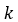number of predictor independent variables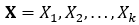, each with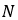rows of data. Then, we would initialize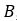, the number of bootstrap models to be fitted, as well as, the number of data rows to use in the bootstrap, where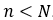. Starting with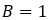, we take a bootstrap resampling ofdata rows and fit the model; specifically, we sample with replacement, to fitand obtain a forecast fit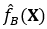. Repeat the process applying a resampling with replacement and generating the aggregate consensus forecast. Recall that this bootstrap approach assumes that the model is correctly specified, and we are simply re-running the same model specification on resampled data. In contrast, the Ensemble Learning methods such as the AI/ML Ensemble Common Fit and the Ensemble Complex Fit will take the same dataset and apply hundreds or even thousands of models to test for the best-fitting model specification.

Bootstrapping works well in situations where the dataset consists of N i.i.d. data points. This means that the sequential order of the data points is not important in fitting the underlying process. For example, if we sufficiently resample rows of data (one row may consist of multiple columns of independent variables) with replacement, the fitted parameters will be distributed around the true population parameters. There might be situations where bootstrap regression is problematic, especially when the data points are not i.i.d. such as when the data points are clumpy or sensitive to extreme values.

Figure 9.55 illustrates the AI Machine Learning Bagging Linear Fit Bootstrap supervised model in BizStats. To get started, the standard practice is to divide your data into training and testing sets. The training set (one dependent with one or more independent variables) is used to train the algorithm and obtain the best-fitting parameters. Note that Risk Simulator also provides a variation of this bootstrap regression by resampling the residual errors as well as randomizing the data and generating probabilistic Monte Carlo simulation assumptions as a result.

The algorithm also allows you to optionally enter known testing set dependent values. Sometimes these are known and sometimes they are unknown and are to be forecasted. If the values are unknown, simply leave the input empty or enter a 0 in the input if you wish to enter the next input, which is the forecast results save location in the data grid.

Simply enter the variables you need to classify and enter the number of clusters desired. For instance, the required model inputs look like the following: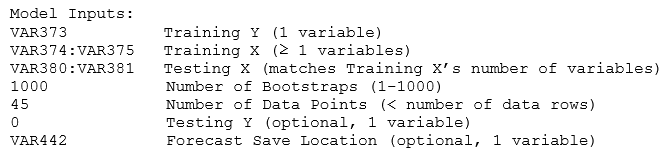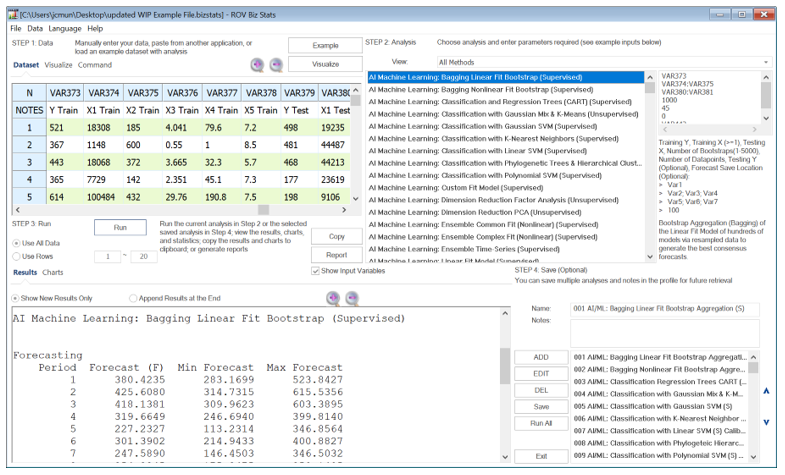Figure 9.55: AI/ML Linear Fit Bootstrap Aggregation or Bagging

Some sample results are shown next. Because there could be hundreds to thousands of bootstrap models, there is no reason to display all the coefficients. The only critical results we care about are the averages of forecast predictions. The interpretations of these forecast values are the same as in multiple linear regression, except that these forecasts are based on the average of hundreds to thousands of bootstrapped regressions to generate a consensus prediction. If you need the fitted coefficients and goodness of fit for the linear fit model, use the AI/ML Linear Fit Model (Supervised) to generate the results of a single model.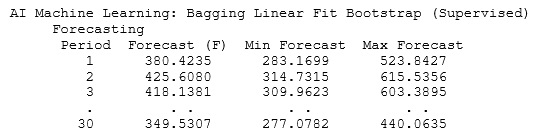error: Content is protected !!